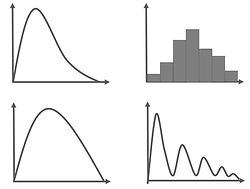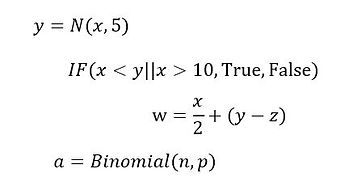# AGENARISK DESKTOP

Bayesian Network Design Software

AgenaRisk Desktop is a design and execution environment for Bayesian Networks which runs on Windows, Linux and Macintosh operating systems. Models developed in AgenaRisk Desktop can then be deployed and shared with other AgenaRisk Desktop users and also used by AgenaRisk Developer and AgenaRisk Enterprise.BAYESIAN NETWORKS (RISK GRAPHS)

• Drag and drop model creation.

• Network composed from nodes (variables) and edges (conditional dependencies).

• Multiple node types: Boolean, Continuous, Labelled, Ranked, Discrete Real.

• Shape, color, text editing.

• Risk graphs and statistics overlay Bayesian network.

• Grouping, zooming, alignment.

• Copy, cut and paste.

• Add, delete and edit multiple scenarios as set of evidence.

• Enter and withdraw soft and hard evidence.

GRAPHS AND STATISTICS

• Risk graphs zoomable and scalable.

• Graphs fixable on Bayesian network or can free float or be pinned.

• Area, histogram, line and point graph types.

• Multiple graph overlays for multiple scenarios, color coded.

• Density and cumulative plots

• Percentile plots.

• Transparency and legends.

• Summary statistics and state value lists.LEARNING FROM DATA

• Tailored Bayesian Networks can be constructed to learn parameters from data (E.g. Multinomial, Normal, Beta-Binomial, Hierarchical models etc).

• Expectation-Maximization algorithm used to learn node probability tables and Gaussian parameters from data.

• Learn from data alone, using EM, and learning from data with expert judgement.

• Handles missing data.

NODE PROBABILITY TABLES

• Conditional probability values entered using node probability table.

• Table cells size can be adjusted for ease of display and navigation.

• Probability values can be cut and pasted from other applications and from within AgenaRisk.

• Conversion of probability tables to/from expressions and partition functions supported.

• Cells automatically normalized.STATISTICAL DISTRIBUTION FUNCTIONS

• Normal and Truncated Normal.

• Beta distribution and Beta PERT.

• Student's t and logistic.

• Chi-square.

• Uniform.

• Exponential, Gamma and Weibull.

• Extreme value and LogNormal.

• Triangular.

• Binomial.

• Hypergeometric.

• Negative Binomial and Geometric.

• Poisson.

FORMULAE EXPRESSION PARSER

• NoisyOR, NoisyAND, mFROMn.

• Arithmetical operators +, -, *, /, ^.

• Comparative expressions, IF(x < 10, "True",....).

• Functions: log( ), ln( ), sqrt( ), e, pi, mod( ), min( ), max( ).

• Comparative operators: Equals ==, Not Equals !=, <, >, >=/ <=, &&, (AND),  || (OR), XOR.

• Ranked node functions: wmean. wmax, wmin, mixminmax.

• Trigonometric functions.

• All functions can be applied with dynamic discretization to identify high density regionsSENSITIVITY AND MULTIVARIATE ANALYZERS

• Sensitivity analysis assesses the impact of a number of variables on one target variable.

• Output Tornado chart in HTML with supporting tables.

• For continuous nodes mean, variance, median, percentile and standard deviation statistics can be analyzed.

• Multivariate analysis queries the model and computes the joint distribution of a user selected set.

• Multivariate analysis output includes statistics and Pearson and rank correlation metrics.

• Multivariate analysis output in CSV file format.

HYBRID INFLUENCE DIAGRAMS

• Identifies max/min expected utility of set of interrelated decisions.

• Conditioned on set of observable and unobservable chance variables.

• Any number of utility nodes combined into a single utility for analysis.

• Utility nodes can be statistical distributions and point values.

• Optimized utility function can be max/min or user defined.

• Output in form of decision tree showing optimum utilities at each branch in the tree and identifying optimal decision set.

• Results exported as HTML with embedded tables/graphs.

• Option available to compute fast results using pre computed static discretization of continuous variables.VALUE OF INFORMATION ANALYSIS

• Determine how much to pay for perfect information prior to making a decision.

• Compute for list of discrete and continuous uncertainty nodes simultaneously.

• Any number of utility nodes combined into a single utility for analysis.

• Expected Value (Partial) Perfect Information computed under each decision option.

• Utility nodes can be statistical distributions and point values.

• Option available to compute fast results using pre computed static discretization of continuous variables.

RISK OBJECT MODELING

• Modular, object based support for model construction using Risk Objects.

• Model import to reuse Risk Objects

• Risk Objects declared with Input and Output nodes as interfaces.

• Statistics can be passed as parameters between Risk Objects as can marginal distribution results.

• Computation performed on whole model or locally on each Risk Object with its ancestors.COMPOUND SUM ANALYSIS

• Compute the compound sum from event frequency distribution and event severity distribution variables, of any type.

• Frequency and severity variables can be conditioned on other variables.

• Resulting compound sum distribution computed using AgenaRisk's own convolution algorithm.

MODEL LIBRARY

• A comprehensive set of example models covered in our book and from applications.

• Examples linked to tutorial material in the AgenaRisk user manual.

• Examples cover: medical diagnosis, legal reasoning, Insurance pricing, stress testing, parameter learning, Six sigma, value of information and decision trees, fault trees, drug economics, business investment....and many more.DATA IMPORT EXPORT

• Data Import via CSV files.

• Data Export via CSV files.

• Model Result Export via CSV files.

• Export Model as XML files.

• Export Statistics as CSV files.

• Export Graphs as JPEG files.

• Analysis results exported as HTML.

ALGORITHMS

• Efficient implementation of Junction Tree algorithm for exact inference in discrete Bayesian Networks

• Dynamic discretization algorithm for approximate inference in Hybrid Bayesian Networks, containing continuous and discrete variables.

• Efficiency improved by automatic binary factorization of expressions and distribution functions in background during computation.

• Hybrid Influence Diagrams algorithm supports hybrid Bayesian Networks; competing methods can only handle point utility values and not distributions.